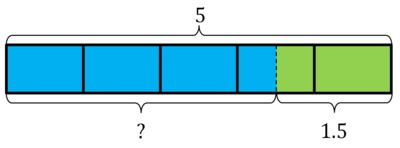##### Engage your students with effective distance learning resources. ACCESS RESOURCES>>

Alignments to Content Standards: 4.MD.A.2

Margie bought 3 apples that cost 50 cents each. She paid with a five-dollar bill. How much change did Margie receive?

## IM Commentary

This task gives students an opportunity to work with familiar fractions and decimals in a context involving money. This task could be used early in the year for formative assessment or as a transition to more complex problems. It also provides a good opportunity to review the connection between familiar fractions and decimals.

Whichever approach students take to solve this problem, they will need to convert between cents and dollars. Working with whole numbers, one dollar is equivalent to 100 cents. Working with fractions 50 cents is $\frac{1}{2}$ of a dollar. Finally, as a decimal, 50 cents is 0.5 dollars. All three approaches are important and students should be comfortable with all of them.

This task was adapted from problem #1 on the 2011 American Mathematics Competition 8 (AMC) Test. For the 2011 AMC 8, which was taken by 153,485 students, the multiple choice answers for the problem had the following distribution:

 Choice Answer Percentage of Answers (A) $1.50 1.78 (B)$2.00 0.54 (C) $2.50 1.81 (D)$3.00 1.30 (E)* $3.50 94.29 Omit -- 0.27 Of the 153,485 students, 72,648 or 47% were in 8th grade; 50,433, or 33% were in 7th grade; and the remainder were in 6th grade or earlier. ## Solutions Solution: 1 Using cents as a unit The apples cost 50 cents each so three apples cost 3$\times$50 cents which is 150 cents. Margie paid 5 dollars. Since one dollar is 100 cents this means that 5 dollars is 5$\times$100 = 500 cents.So Margie will get back $$500 - 150 = 350 \,{\rm cents}.$$ This is the same as \$3.50.

Solution: 2 Using dollars and fractions

The apples cost 50 cents each. This is $\frac{1}{2}$ of a dollar. So two apples will cost 1 dollar and 3 apples cost an extra $\frac{1}{2}$ dollar making 1$\frac{1}{2}$ dollars. Margie paid 5 dollars.So Margie will get back $$5- 1\frac{1}{2} \,{\rm dollars}.$$ Taking away one dollar leaves Margie with 4 dollars and taking away an additional half dollar means Margie will get back 3$\frac{1}{2}$ dollars or \$3.50. Solution: 3 Using dollars and decimals from the start The apples cost 50 cents each. Since one cent is 0.01 dollars, 50 cents is$0.50\$ dollars. Two apples will cost 1 dollar and 3 apples cost an extra 50 cents making 1.50 dollars. Margie paid 5 dollars. So Margie will get back $$5- 1.50 \,{\rm dollars}.$$ Taking away one dollar leaves Margie with 4 dollars and taking away an additional 0.50 dollars means Margie will get back 3.50 dollars.